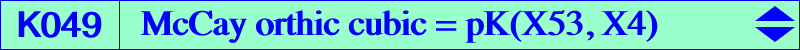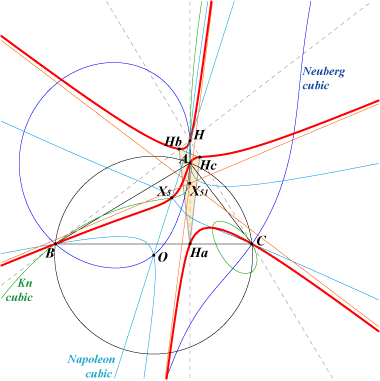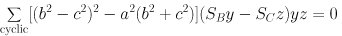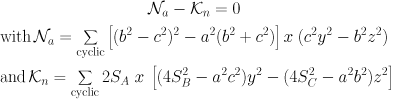X(4), X(5), X(52), X(847) infinite points of the McCay cubic points of pK(X6, X52) on (O) vertices of orthic triangle i.e. feet of altitudes traces of the cevian lines of X(847) on the orthic triangle sidelines imaginary foci of the Brocard ellipse i.e. common points of the Brocard axis and the Kiepert hyperbola Ix-anticevian points : see Table 23 X550-OAP points, see also Table 53.This is the McCay cubic of the orthic triangle. We meet it in Special isocubics §6.6.1. It is a member of the class CL006 of pK60+ cubics. See also the related K627, CL049 and Table 51. It is a pK60+ with pole X(53), pivot X(4). Its three real asymptotes are parallel to those of the McCay cubic and concur at X(51) = X(2)-orthic. K049 is tangent at X(4) to the Euler line and tangent at A, B, C to the cevian lines of X(5). K049 is a member of the pencil of stelloids generated by the McCay cubic and the union of the line at infinity with the Kiepert hyperbola. K049 also belongs to the pencil of cubics D(k) containing the Napoleon cubic, the Kn cubic. This is D(1) in "Two Remarkable Pencils..." in the Downloads page. Its equation rewrites as :The isogonal transform of K049 is K373 = pK(X14533, X54). K049 is spK(X3, X389) as in CL055. K957 is the Hessian of K049.